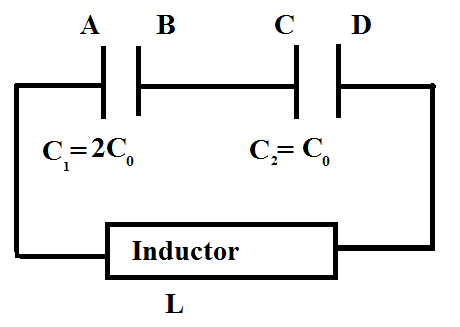Inductor + CapacitorsTwo capacitors of capacitances $C_1$ and $C_2$ are connected in series with an inductor of inductance $L$. Initially the capacitors have charge such that $V_B-V_A=4V_0$ across $C_1$ and $V_C-V_D=V_0$ across $C_2$. Now if

a) Maximum current in circuit is $\xi$.

b) Potential drop across $C_1$ is $\mu$ at that instant.

c) Potential drop across $C_2$ is $\eta$ at that instant.

d) Equation of current flowing towards left in the inductor is given by $i= \psi \left( \sin { \left( \frac { \omega t }{ \varsigma } \right) } \right)$.

Find $\xi +\mu +\eta +\psi +\omega +\varsigma$.

Details And Assumptions:

• $C_0=2F$

• $L=\dfrac 13 \ H$

• $V_0=4 \ \text{volt}$

• Initially the current in the circuit is zero.

• $\omega$ and $\varsigma$ are co-prime.

×